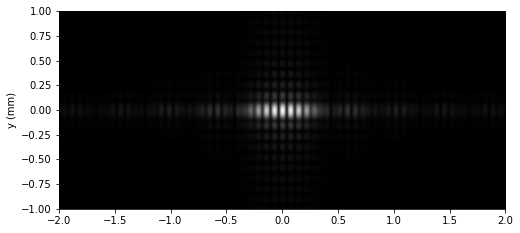## Simulating Light Diffraction with Lenses - Visualizing Fourier Optics

Most interesting and practical optical instruments use lenses to manipulate the formation of images, so understanding how lenses affect the propagation of light is critical to understand Fourier optics.
In the above video, we'll illustrate some of the principles of Fourier optics visually and intuitively by showing how lenses affect …

## Simulating Diffraction Patterns with the Angular Spectrum Method and Python

In this project we will show how to numerically compute Diffraction Patterns with the Angular Spectrum Method. We'll implement the method with Python and discuss how to simulate them both with monochromatic and polychromatic light like it's shown in the video above.

## The Angular Spectrum

In a previous post we …

## Visual Explanation of the Van-Cittert Zernike Theorem - The Double Slit Experiment with Incoherent and Coherent Light

What happens when the double slit experiment is performed with incoherent light (for example with a light bulb)? And how it differs when it is performed with coherent light (for example with a laser)?

The main idea of these simulations is to answer this question, simulating the light propagating through …

## Solving the Diffraction Integral with the Fast Fourier Transform (FFT) and PythonIn this project we will show how to numerically compute the Fresnel Diffraction Integral with the Fast Fourier Transform (FFT). We'll implement the method with Python and we will apply it to the study of the diffraction patterns produced by the particle beams in the double slit experiment, showing the …

© 2019-2022 Rafael de la Fuente